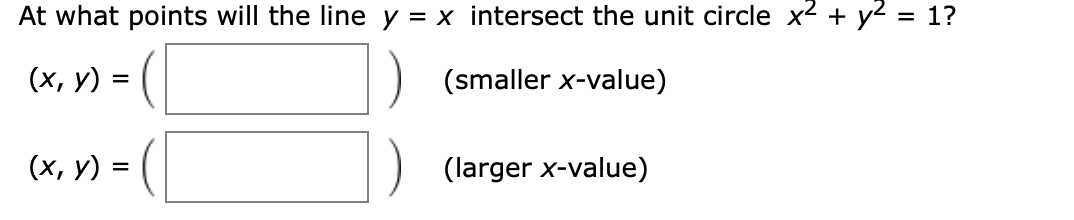# At what points will the line y = x intersect the unit circle x2y = 1?(х, у)(smaller x-value)(х, у) %3(larger x-value)

Question
20 views

at what point do the line intersect on the unit circlehelp_outlineImage TranscriptioncloseAt what points will the line y = x intersect the unit circle x2y = 1? (х, у) (smaller x-value) (х, у) %3 (larger x-value) fullscreen
check_circle

Step 1

The given line is,

Step 2

The equation of the unit circle is,

Step 3

Solve the two equations to obtain the points at which ...

### Want to see the full answer?

See Solution

#### Want to see this answer and more?

Solutions are written by subject experts who are available 24/7. Questions are typically answered within 1 hour.*

See Solution
*Response times may vary by subject and question.
Tagged in

### Other